Function Repository Resource:

# ElevateGeoPosition

Set the elevation of one or multiple geodetic positions

Contributed by: Jesse Friedman
 ResourceFunction["ElevateGeoPosition"][pos,value] return geodetic position pos with its elevation set to value. ResourceFunction["ElevateGeoPosition"][pos,f] apply f to each elevation value in pos. ResourceFunction["ElevateGeoPosition"][{pos1,pos2,…},…] adjust the elevation of each of the posi. ResourceFunction["ElevateGeoPosition"][value] represents an operator form of ResourceFunction["ElevateGeoPosition"] that can be applied to an expression.

## Details and Options

pos can be a GeoPosition or GeoGridPosition object, or an entity with an associated geographic position.
value can be a numeric object (assumed to be in meters) or a Quantity expression.
With ResourceFunction["ElevateGeoPosition"][pos,f], f is applied to the elevation component of each point in pos. Any absent elevation components are taken to have a geodetic elevation of 0 meters.
ResourceFunction["ElevateGeoPosition"][entity,] is equivalent to ResourceFunction["ElevateGeoPosition"][GeoPosition@entity,].
ResourceFunction["ElevateGeoPosition"][value][pos] is equivalent to ResourceFunction["ElevateGeoPosition"][pos,value].

## Examples

### Basic Examples (3)

A point 100 meters above the reference ellipsoid at the current geo location:

 In:=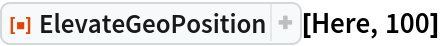Out=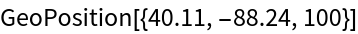Set the elevation of each member of a geo position array:

 In:=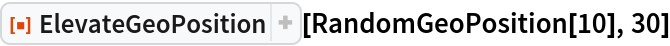Out=In:=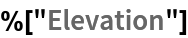Out=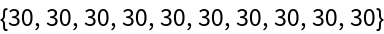Get a GeoPosition representing the summit of Olympus Mons:

 In:=Out=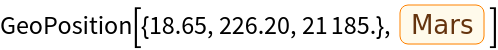### Scope (4)

GeoPosition objects of arbitrary dimensions are supported:

 In:=Out=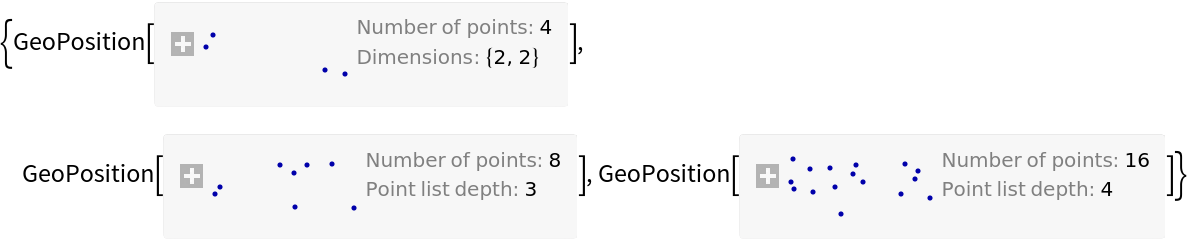In:=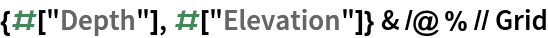Out=GeoGridPosition objects are supported:

 In:=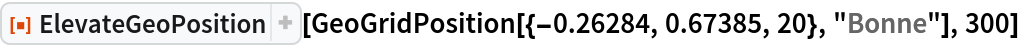Out=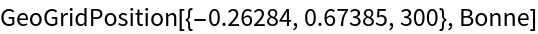Add 100km to the current elevations of each of the GPS satellites by supplying a transformation function:

 In:=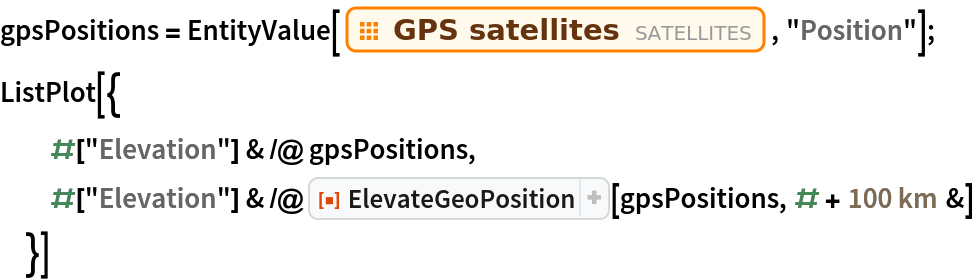Out=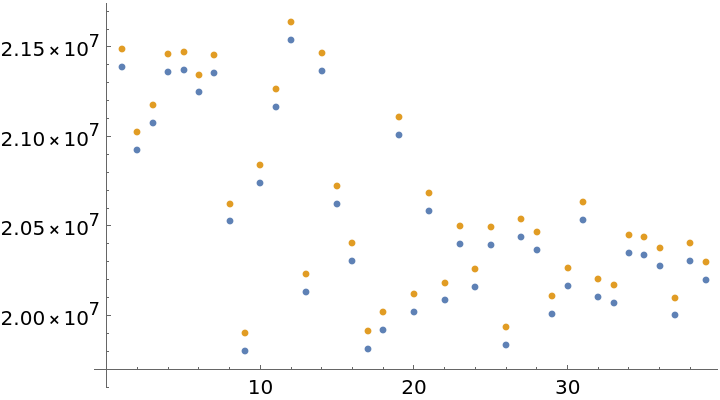An operator form of ElevateGeoPosition is available:

 In:=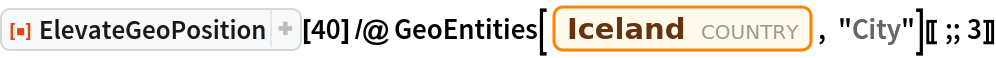Out=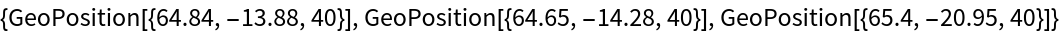### Possible Issues (2)

GeoPositionXYZ and GeoPositionENU objects are not supported, as they do not directly encode geodetic elevation:

 In:=Out=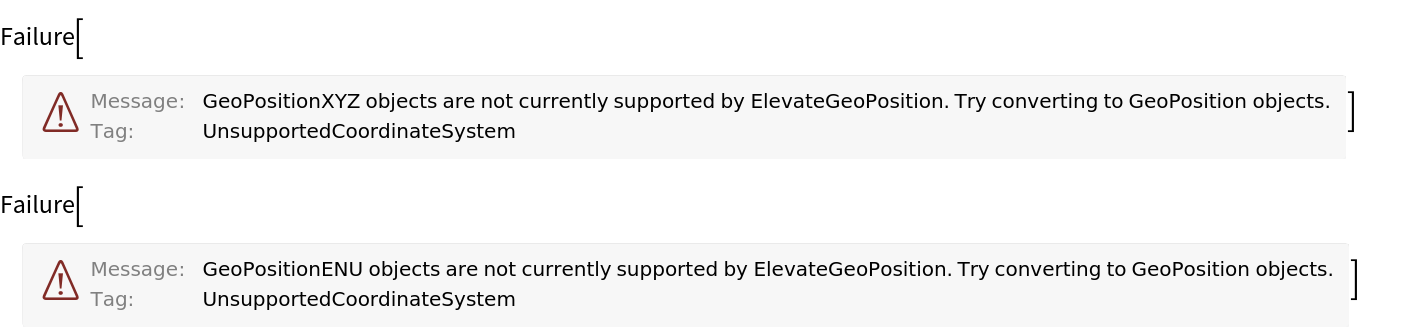These positions can be used by converting them to GeoPosition objects before applying ElevateGeoPosition, and back to their original coordinate systems after:

 In:=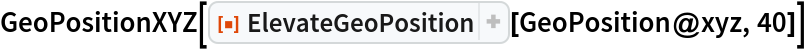Out=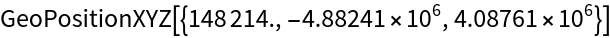In:=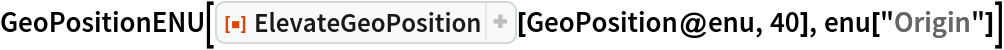Out=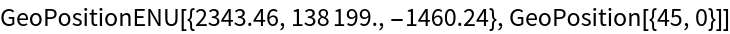Jesse Friedman

## Version History

• 1.0.0 – 22 October 2019